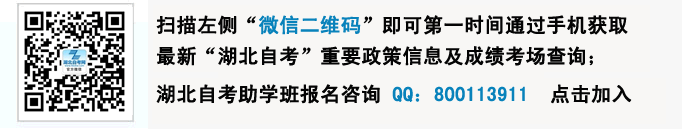# 自考计算机基础与程序设计试题_全国2009年4月自考试卷

 湖北自考新旧专业转接通知 2021年10月自考报考简章 2021年10月湖北自考计算机化考试报名 2021年10月湖北自考考试时间 2021年10月自考教材大纲 湖北自考电子档案查询系统入口 湖北省高等教育自学考试考生服务平台 自考毕业申请指导手册 2021年学位英语网络课程，提高通过率 湖北自考新手报名指导手册 湖北历年自考试题 湖北自考各专业介绍

1.衡量存储器的基本单位是（    ）
A. bit B. byte
C. KB D. word
2.Windows操作系统是（    ）
A. 单用户、单任务 B. 单用户、多任务
C. 多用户、单任务 D. 多用户、多任务
3.组成微机主存储器的是（    ）
A. CD—ROM和RAM B. 硬盘和RAM
C. 硬盘和CD—ROM D. ROM和RAM
4.下列数据中最大数是（    ）
A. （1010010）2 B. （123.4）8
C. (84.5)10 D. (55)16
5.在Turbo C 2.0中，运行一个程序后，要查看运行结果可按键（    ）
A. Alt+F1 B. Alt+F5
C. Alt+F9 D. Alt+F10
6.下列是合法C语言常量的是（    ）
A. e B. 7E4.0
C. E4 D. -9.13E-30
7.设有int  a=3,b=-4,c=5;表达式（a<b）?a:b&&c<0的值是（    ）
A. 0 B. 1
C. 3 D. 4
8.以指数形式输出实型数的格式说明符是（    ）
A. %u B. %x
C. %f D. %e
9.下列程序的输出结果是（    ）
main(  )
{ int  x=5,y;
if(x<0)y=-1;
if(x==0)y=0;
y=1;
printf(″%d″,y);
}
A. -5 B. -1
C. 0 D. 1
10.下列程序段的输出结果是（    ）
i=1;
while(i<3){
j=1;
while(j<=i){
printf(″%d″,i+j);
j++;
}
i++;
printf(″\n″);
}
A. 2 3 3 4 B. 2 3 4
C. 2 3  D.2
3 4                                3 4
11.已知str1和str2是字符数组名，下面函数中能正确地完成字符串输出的是（    ）
A. puts (str1,str2) B. puts (str2)
C. putchar (strl) D. putchar (str1,str2)
12.下列关于函数的说明中不正确的是（    ）
A. 函数名后面可以不要一对圆括号
B. 函数参数类型必须作说明
C. 函数形参个数可以是一个、多个或没有
D. 空函数被调用时不做任何工作
13.若有定义：int  a;则a数组中首元素的地址可以表示为（    ）
A. &a B. a+1
C. a D. &a
14.设有char     s=″\ta\017bc″;则指针变量s指向的字符串在内存中所占的字节数是
（    ）
A. 5 B. 6
C. 7 D. 9
15.下列关于结构体类型的定义正确的是（    ）
A. struct  tree B. struct  tree
{  int  branch;                     {  int branch;
char  name; char  name,
char    relation; char    relation,
}; };
C. struct  tree D. struct  tree
{  int  branch                     {  int  branch;
char  name char  name;
char    relation char    relation;
} }
16.下列各个位运算符的优先级从左到右依次降低的是（    ）
A. | & ˆ > > B. ˆ & > > |
C. ～ > > ˆ | D. ～ > > | ˆ
17.设有说明:int  u=1,v=3,w=5;表达式：v &～～u|w的值是（    ）
A. 3 B. 5
C. 6 D. 8
18.设有下列程序：（    ）
#include″stdio.h″
main(  )
{
unsigned  x=8,y=2;
printf(″%d\n″,y|～(x&y));
}

A. -1 B. 0xff
C.127  D. 65535
19.若有定义∶# define  P(x)  x   x  x+1和说明语句：int  a=2;则表达式P（2）的值是（    ）
A. 6 B. 8
C. 9 D. 10
20.在语句fgets(str,n,p);中，下列说法不正确的是（    ）
A. str是字符指针 B. 一次可以读n个字符
C. str是字符数组名 D. 一次可以读n-1个字符

21.下列关于计算机的叙述中正确的是（    ）
A. CPU是计算机系统的部件
B. I/O设备不是计算机系统的部件
C. 计算机区别于其他计算工具的本质特点是能存储数据
D. “裸机”是指不配置任何软件的计算机
E. 计算机(武汉自考)指令是控制计算机进行操作的命令
22.设有int  a=3,b=-5;下列表达式的值等于1的有（    ）
A. a&&b<0 B. fabs(b)-a-1>0
C. a>b&&b<0 D. (a,2,-5)==(b,2,a)
E. a+b<0
23.下列关于break、goto、continue语句的说法中正确的是（    ）
A. break语句能够用于循环语句中
B. break语句不能用于switch语句中
C. goto语句可以用于直接从多层循环中退出
D. continue语句可用于跳出循环体
E. continue语句不能用于switch语句中
24.若有定义:int  y,(  p);及赋值p=y;则下列语句中可以输出数组元数y的值的语句是（    ）
A. printf (″%d\n″,&y); B. printf (″%d\n″, y);
C. printf (″%d\n″,p); D. printf (″%d\n″, y);
E. printf (″%d\n″,   (  (y+2));
25.设有关于结构体类型的定义：
struct  worker
{
int  id;
char    name;
float  salary;
} wl={102035,″Lihua″,2560.50},  p;

A. p=wl; B. p=&wl;
C. p->id=wl->id D. p->id=wl.id;
E. p.salary=wl.salary;

26.有无default对switch语句有什么不同的影响？
27.写出do-while语句的一般形式及其执行过程。
28.定义 char  a[  ]=″I am a student.″,   str=a;等价于
char  a[  ]= ″I am a student.″,   str;
str=a;

29.设有说明：
struct  vegetable
{
char    part;
char    color;
} clove;
char    part _ of _ vegetable ={″AA″,″BB″，″CC″}；
char    seven _ color={″11″,″22″,″33″,″44″,″55″,″66″,″77″};

30.阅读分析下面程序后，写出程序的运行结果。
# include ″stdio.h″
main (  )
{
int  a=978,x,y,z,i,j,w；
x=a/100;
y=(a-100   x)/10;
z=a-10  y-100   x;
i=y>z? z:y;
j=y<=z? z:y;
w=100   x+10   i+j;
printf(″%d,%d,%d,%d\n″,x,y,z,w);
}
31.阅读分析下面程序后，写出程序的运行结果。
# include ″stdio.h″
main(  )
{
int  n,m;
for(n=1;n<=5;n++)
{
for(m=1;m<=4;m++)
printf(″%4d″，m   n);
printf(″\n″);
}
}
32.阅读分析下面程序后，写出程序的运行结果。
#include″stdio.h″
main (  )
{
int  arr,i,k=0;
for(i=0;i<10;i++)
arr[i]=i;
for(i=1;i<=4;i++)
{
k+=arr[i]+i;
printf(″%5d″,k);
}
}
33.阅读分析下面程序后，写出程序的运行结果。
#include″stdio.h″
int  fun(int  x)
{
int  p;
if(x==0||x==1)
return(3);
p=x-fun(x-2);
printf(″%5d″,p);
return  (p);
}
main(  )
{
fun(9);
}
34.阅读下面程序，分析cat函数的功能并写出程序的运行结果。
#include″stdio.h″
void  cat(char    s,char    t)
{
while( s)  s++;
while((  s++=  t++)!=′\0′);
}
main(  )
{
char  sl=″computer″,s2=″Pentium_″;
cat(s2,s1);
printf(″% s\n″,s2);
}
35.阅读分析下面部分程序：
struct  cjd
{
char  name;
float  score;
}  x;
float  t={70.5,65.0,83.0,92.5};
int  j,k;

36.请编程从键盘上输入十个整数，计算其中大于零的奇数的平均值，并输出这些大于零的奇数以及它们的平均值。
37.有若干个学生（不超过50人）的某一门课的成绩放在文件c:\cj.dat中，要求编程统计出90～100分，80～89分，70～79分，60～69分，0～59分的人数各有多少人。#### 湖北自考考生微信交流群